#How oversampling yielded great results for classifying cases of Sexual Harassment.

#### The problem: Overcoming an imbalanced data set

When it comes to data science, sexual harassment is an imbalanced data problem, meaning there are few (known) instances of harassment in the entire dataset.

An imbalanced problem is defined as a dataset which has disproportional class counts. Oversampling is one way to combat this by creating synthetic minority samples.

#### The power of oversampling

SMOTE — Synthetic Minority Over-sampling Technique — is a common oversampling method widely used in machine learning with imbalanced high-dimensional datasets using Oversampling. The SMOTE technique generates randomly new examples or instances of the minority class from the nearest neighbors of a line joining the minority class sample to increase the number of instances. SMOTE creates synthetic minority samples using the popular K nearest neighbor algorithm.

K nearest neighbors draw a line between the minority points and generate points in the middle of the line. It is a technique that was experimented on, nowadays one can find many different versions of SMOTE which build upon the classic formula. Let’s visualize how oversampling effects the data in general.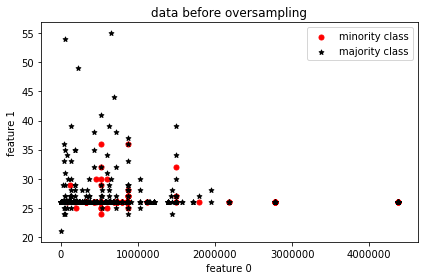Visual representation of data without oversampling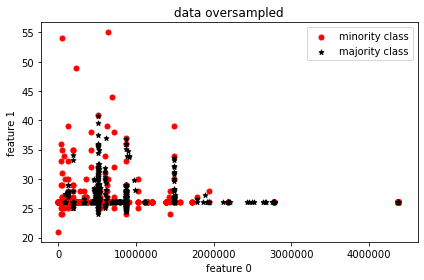Visual representation of data with oversampling

For visualization’s sake, two features are picked and from their distribution, it’s clearly seen that the minority samples match the majority sample count.

#### Impact on the predictions

Let’s compare the predictive power of oversampling vs. not oversampling. Random Forest is used as the predictor in both cases. The ProWSyn version of oversampling is selected as the highest performing oversampling method after all the methods are compared using this Python package.

Let’s check the performance of models pre and post oversampling.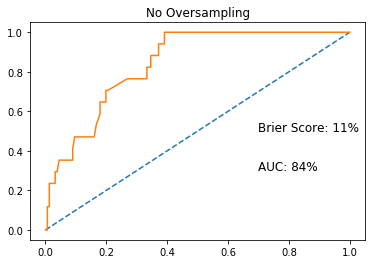ROCAUC without oversampling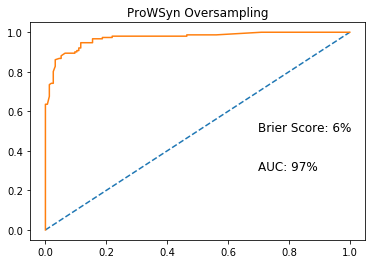ROCAUC with oversampling

With ProWSyn oversampling implemented, we can see a 13% increase in the ROCAUC score, which is the Area Under the Receiver Operating Characteristics curve, from 84% to 97%. I was also able to decrease the Brier Score, which is a metric for probability prediction, by 5%.

As you can see from the results, oversampling can significantly boost your model performance when you have to deal with imbalanced datasets using oversampling. In my case, the ProWSyn version of SMOTE performed the best but this depends always on the data and you should try different versions to see which one works the best for you.

#### What is ProWSyn and why does it work so well?

Most Data Science: oversampling methods lack a proper process of assigning correct weights for minority samples, in this case regarding the classification of Sexual Harassment cases. This results in a poor distribution of generated synthetic samples. Proximity Weighted Synthetic Oversampling Technique (ProWSyn) generates effective weight values for the minority data samples based on the sample’s proximity information, i.e., distance from the boundary which results in proper distribution of generated synthetic samples across the minority data set.

#### What is the output?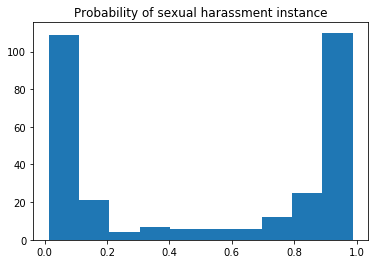x: number of instances; y: probability

After the prediction, the histogram of predicted probabilities looks like the image above. The distribution turned out the be the way I imagined. The model has learned from the many features and it turns out there is a correlation within the feature space which at the end creates such a distinct difference between classes 0 and 1. In simpler terms, there is a pattern within 0 and 1 classes’ features.

More care has to be put into probabilities really close to 1 (100% probability). From the histogram plot above, we can see that the number of points near 100% probability is quite high. It is normal to dismiss someone as a non-predatory but much harder to accuse someone, therefore that number should be lower.

Original post can be viewed here

Views: 1036

Comment

Join Data Science Central